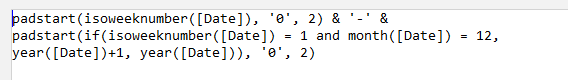# Date from isoweeknumber

Hello,

i want to calculate a date only from the year and isoweeknumber. For my example i use year 2018 and KW47. How can i get a date out of that two values?

Regards,
Michael

I understand that you will have to find the 1st Monday of year 2018 and then add 47 weeks. See the example below:

isoweeknumber2date.morph (2.0 KB)

1 Like

Thank´s DmitryYou’re welcome!1. Is there a solution to format the Isoweek with two digits (01-52)?
2. 31.12.2018 ist week 1 in 2019 how can the value be determined? Is there a Function like weekjear?

You can use thepadstart() function to add leading zeros to the result of the isoweeknumber() function. E.g.:

``padstart(isoweeknumber(#2018-12-31), '0', 2) //returns "01"``

There is no function weekyear() in EasyMorph, however you can use the expression below as an equivalent:

``padstart(isoweeknumber([Date]), '0', 2) & '-' & padstart(if(isoweeknumber([Date]) = 1 and month([Date]) = 12, year([Date])+1, year([Date])), '0', 2)``

Hi

Just want to add, if you’re talking ISO week, you should also talk ISO year (year of Thursday?).
With current logic, at New Year 20/21, you will have 53-2021 in the beginning of 2021, while the first dates are in fact related to 53-2020. - See below.

I also made a version of the week/year calculation - taking year first and applying week at the end for continous sorting.

Original Week-Year calculationNew YearWeek calculation
`if(weekday([Date])=1,year(weekstart([Date])-7+4)*100+isoweeknumber([Date]),year(weekstart([Date])+4)*100+isoweeknumber([Date]))`

1 Like

Thanks for the correction, @DKcrm.